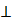SSC BOARD PAPERS IMPORTANT TOPICS COVERED FOR BOARD EXAM 2024

### Practice Set 5.3, Chapter 5 - Quadrilaterals Mathematics Part II Solutions for Class 9 Math

Practice Set 5.3
###### Practice Set 5.3
Question 1.

Diagonals of a rectangle ABCD intersect at point O. If AC = 8 cm then find BO and if ∠CAD = 35° then find ∠ACB.

The diagonals of a rectangle are congruent to each other and bisects each other at the point of intersection so since AC = 8 cm

⇒ BD = 8 cm and

O is point of intersection so DO = OB = AO = OC = 4 cm

⇒ ∠ACB = 35 °

(since AB ∥ DC and AC is transversal ∴ ∠CAD and ∠ACB are pair of alternate interior angle.)

Question 2.

In a rhombus PQRS if PQ = 7.5 then find QR. If ∠QPS = 75° then find the measure of ∠PQR and ∠SRQ.

⇒ all the sides are congruent /equal

⇒ PQ = QR = 7.5

Also ∠QPS = 75° (given)

⇒∠QPS = 75° (opposite angles are congruent)

But ∠QPS + ∠PQR = 180° (adjacent angles are supplementary)

⇒ ∠PQR = 105°

∴ ∠SRQ = 105° (opposite angles)

Question 3.

Diagonals of a square IJKL intersects at point M, Find the measures of ∠IMJ, ∠JIK and ∠LJK.

The given quadrilateral is a square

⇒ all the angles are 90°

∴ ∠JIK = 90°

Since the diagonals areto each other ∠IMJ = 90°

Since the diagonals os a square are bisectors of the angles also

∠LJK = ∠IJL = 1/2 × 90° = 45°

Question 4.

Diagonals of a rhombus are 20 cm and 21 cm respectively, then find the side of rhombus and its perimeter.

Let the diagonal AC = 20cm and BD = 21

AB2 = BO2 + AO2

AB2 = (10.5)2 + (10)2

(the diagonals of a rhombus bisect each other at 90°)

AB2 = 110.25 + 100

AB = √210.25 = 14.5cm (side of the rhombus)

Perimeter = 4a = 14. 5 × 4 = 58cm

Question 5.

State with reasons whether the following statements are ‘true’ or ‘false’.

(i) Every parallelogram is a rhombus.

(ii) Every rhombus is a rectangle.

(iii) Every rectangle is a parallelogram.

(iv) Every square is a rectangle.

(v) Every square is a rhombus.

(vi)Every parallelogram is a rectangle.

(i) False.

Explanation: Every Parallelogram cannot be the rhombus as the diagonals of a rhombus bisects each other at 90° but this is not the same with every parallelogram. Hence the statement if false.

(ii) False.

Explanation: In a rhombus all the sides are congruent but in a rectangle opposite sides are equal and parallel. Hence the given statement is false.

(iii) True.

Explanation: The statement is true as in a rectangle opposite angles and adjacent angles all are 90°. And for any quadrilateral to be parallelogram the opposites angles should be congruent.

(iv) True.

Explanation: Every square is a rectangle as all the angles of the square at 90° , diagonal bisects each other and are congruent , pair of opposite sides are equal and parallel . Hence every square is a rectangle is true statement.

(v) True.

Explanation: The statement is true as all the test of properties of a rhombus are meet by square that is diagonals are perpendicular bisects each other , opposite sides are parallel to each other and the diagonals bisects the angles.

(vi) False.

Explanation:

Every parallelogram is a rectangle is not true as rectangle has each angle of 90° measure but same is not the case with every parallelogram.